#Quadratic Equations : Basic DefinitionsA quadratic function is any function equivalent to one of the form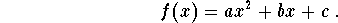Here are some examples of quadratic functions

•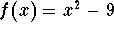•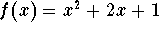•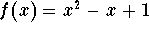•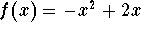A quadratic equation is any equation equivalent to one of the form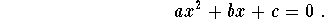Here are some examples of quadratic equations

•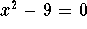•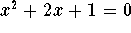•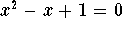•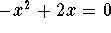[Algebra] [Complex Variables]
[Geometry] [Trigonometry ]
[Calculus] [Differential Equations] [Matrix Algebra]S.O.S MATHematics home page

Do you need more help? Please post your question on our S.O.S. Mathematics CyberBoard.Author: Mohamed Amine Khamsi